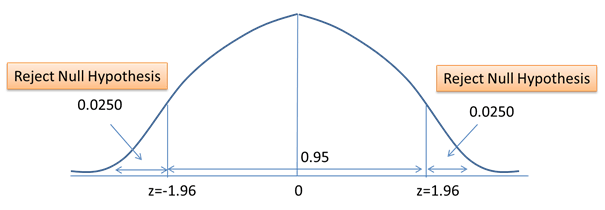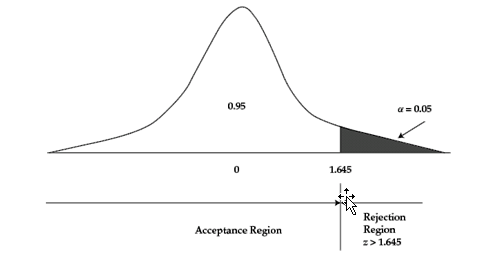# Decision Rule in Hypothesis Testing

A decision rule is the rule based on which the null hypothesis is rejected or not rejected.

We first state the hypothesis. Then we determine if it is a one-tailed or a two tailed test. We then specify a significance level, and calculate the test statistic. Now we calculate the critical value. If the test statistic follows a normal distribution, we determine critical value from the standard normal distribution, i.e., the z-statistic. Using the test statistic and the critical value, the decision rule is formulated.

For a 5% level of significance, the decision rules look as follows:

1. H0: θ = θ0 versus Ha: θ ≠ θ0

Reject the null hypothesis if test-statistic > 1.96 or if test-statistic < -1.96.

2.  H0: θ ≤ θ0 versus Ha: θ > θ0

Reject the null hypothesis if test-statistic > 1.645

3. H0: θ ≥ θ0 versus Ha: θ < θ0

Reject the null hypothesis if test-statistic < -1.645

In our example, the decision rule will be as follows:

Reject the null hypothesis if test-statistic > 1.96 or if test-statistic < -1.96.Our value of test-statistic was 4, which is greater than 1.96. Therefore, null hypothesis should be rejected. This was a two-tailed test.

The following chart shows the rejection point at 5% significance level for a one-sided test using z-test.### Power of Test

The power of test is the probability of correctly rejecting the null (rejecting the null when it is false).

The power of test = 1 – P(Type II Error)

The following table illustrates the correct decision, Type I error and Type II error.

 H0 is true H0 is false H0 is rejected Type I ErrorSignificance level, a. Probability of Type I error. Correct H0 is not rejected Correct Type II ErrorPower of Test. 1 – P(Type II Error)

It is difficult to control for the probability of making a Type II error. Statisticians avoid the risk of making a Type II error by using “do not reject _H_0” and not “accept _H_0.

### Statistical Result Vs Economically Meaningful Result

When we use a hypothesis test to reject a null hypothesis, we have results that are statistically significant. However, this does not necessarily mean that the results are meaningful economically.

For example, our hypothesis may statistically prove that a certain strategy produces returns consistently above the benchmark. However, it does not mean that when we implement that strategy, we will get economically meaningful returns above the benchmark. While implementing we will have to consider many other factors such as taxes, and transaction costs.

Get our Data Science for Finance Bundle for just $29$51.